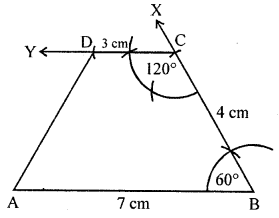## ML Aggarwal Class 8 Solutions for ICSE Maths Chapter 14 Constructions of Quadrilaterals Objective Type Questions

Higher Order Thinking Skills (Hots)
Question 1.
Construct a trapezium ABCD in which AB = 7 cm, BC = 4 cm, CD = 3 cm, ∠B = 60° and AB || DC.
Solution:
Steps of construction :
(i) Draw a line segment AB = 7 cm.
(ii) At B, draw a ray BX making an angle of 60°
and cut off BC = 4 cm.
(iii) At C draw a line CY parallel to AB
making an angle of 120° and cut off CD = 3 cm.
ABCD is the required trapezium.Question 2.
Construct a parallelogram PQRS in which PQ = 6 cm, ∠Q = 120° and ∠RPQ = 30°.
Solution:
Steps of construction :
(i) Draw a line segment PQ = 6 cm.
(ii) At P, draw a ray making an angle of 30° and at Q
a ray making an angle of 120° intersecting each other at R.(iii) With centre P and radius QR and with centre R
and radius equal to PQ, draw arcs intersecting each other at S.
(iv) Join SP and SR.
PQRS is a required parallelogram.

Question 3.
Construct a rectangle ABCD in which diagonal AC = 7 cm and the diagonals BD and AC intersect at O such that ∠BOC = 45°.
Solution:
Steps of construction :
(i) Draw AO= $$\frac{1}{2}$$AC = ($$\frac{1}{2}$$ × 7) cm = 3·5 cm and produce AO to C
such that OC = OA = 3·5 cm.
(ii) At O, construct ∠COP = 45°.
(iii) From OP, cut off OD = $$\frac{1}{2}$$ AC = 3·5 cm.
produce DO to B such that OB = OD = 3·5 cm.
(iv) Join AB, BC, CD, DA. Then ABCD is the required rectangle.